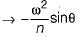Courses

# Test: Static & Dynamic Force Analysis - 2

## 8 Questions MCQ Test Topicwise Question Bank for Mechanical Engineering | Test: Static & Dynamic Force Analysis - 2

Description
This mock test of Test: Static & Dynamic Force Analysis - 2 for Mechanical Engineering helps you for every Mechanical Engineering entrance exam. This contains 8 Multiple Choice Questions for Mechanical Engineering Test: Static & Dynamic Force Analysis - 2 (mcq) to study with solutions a complete question bank. The solved questions answers in this Test: Static & Dynamic Force Analysis - 2 quiz give you a good mix of easy questions and tough questions. Mechanical Engineering students definitely take this Test: Static & Dynamic Force Analysis - 2 exercise for a better result in the exam. You can find other Test: Static & Dynamic Force Analysis - 2 extra questions, long questions & short questions for Mechanical Engineering on EduRev as well by searching above.
QUESTION: 1

Solution:
QUESTION: 2

### A couple M of magnitude 1.5 kN-m is applied to the crank of the engine system shown in figure below. What is the force P required to hold the system in equilibrium?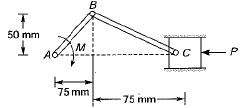Solution: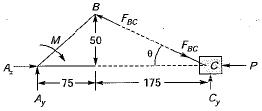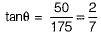FBD of whole engine system: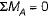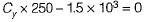∴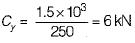FBD of piston: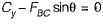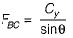Now,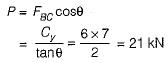QUESTION: 3

### Rod CD is attached to the collar D and passes through a collar welded to end B of level AB. Neglecting the effect of friction, what is the couple M required to hold the system in equilibrium when θ = 300? (AB ⊥ CD and point D and A are collinear)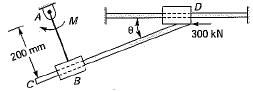Solution:

FBD of DC: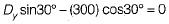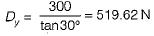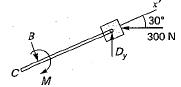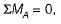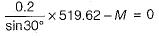∴ M = 207.85 N-m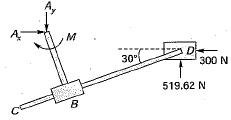QUESTION: 4

A body of mass m and radius of gyration K is to be replaced by two masses m1 and m2 located at distances h1 and h2 from the C.G. of original body. These will be dynamically equivalent to original body. If

Solution:

Conditions for dynamically equivalence:
1. The sum of their masses is equal to the total mass of the body i.e., m = m1 + m2.
2. The centre of gravity of two masses coincide with that of the body.
3. The sum of mass moment of inertia of the masses about their centre of gravity is equal to the mass moment of inertia of the body.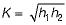QUESTION: 5

When the crank is at the inner dead centre in a horizontal reciprocating steam engine then the velocity of the piston will be

Solution:
QUESTION: 6

Acceleration of the piston in a reciprocating engine is maximum at dead centre and is given by

Solution:
QUESTION: 7

A disc type flywheel having a mass of 10 kg and radius 0.2 m is replaced in a single cylinder engine by a system of dynamically equivalent concentrated masses m1 and m2 rotating about the fly wheel axis as shown below, if the distance x1 is 0.1 m then the distance x2 is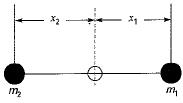Solution:

Condition for dynamically equivalent system,
x1x2 = k2
where k = radius of flywheel
∴ 0.1 x x2 = (0.2)2
⇒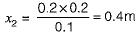QUESTION: 8

For a slider-crank mechanism with radius of crank r length of connecting rod I, obliquity ratio, crank rotating at an angular velocity ω; for any angle q of the crank, match List-I (Kinematic Variable) with List-II (Equation) and select the correct answer:
List-1
A. Velocity of piston
B. Acceleration of piston
C. Angular velocity of connecting rod
D. Angular acceleration of connecting rod
List-II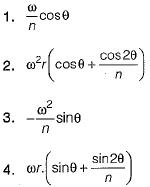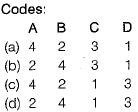Solution:

1. Velocity of piston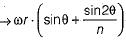2. Acceleration of piston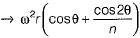3. Angular velocity of connecting rod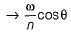4. Angular acceleration of connection rod